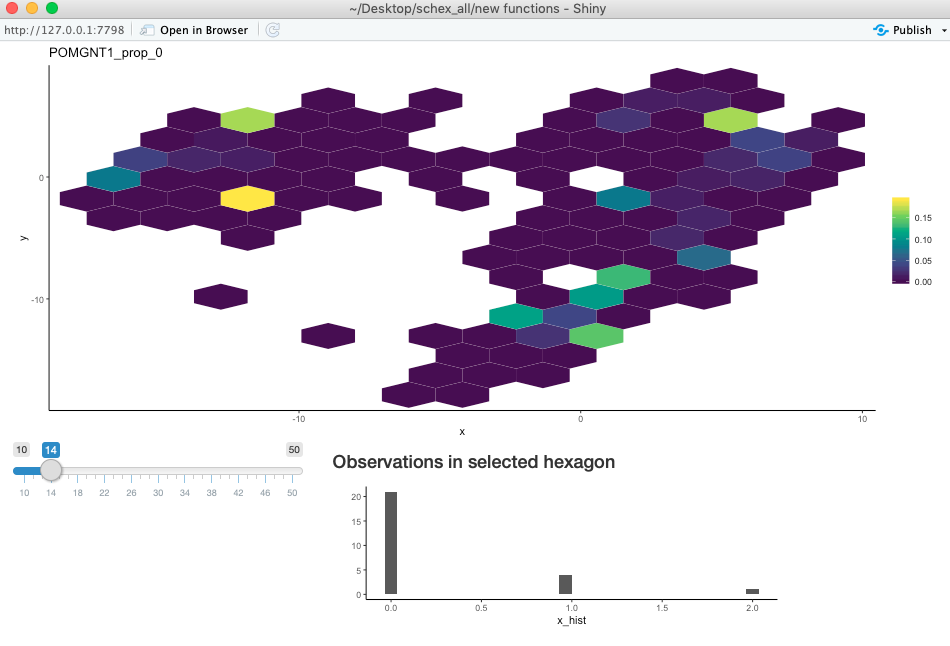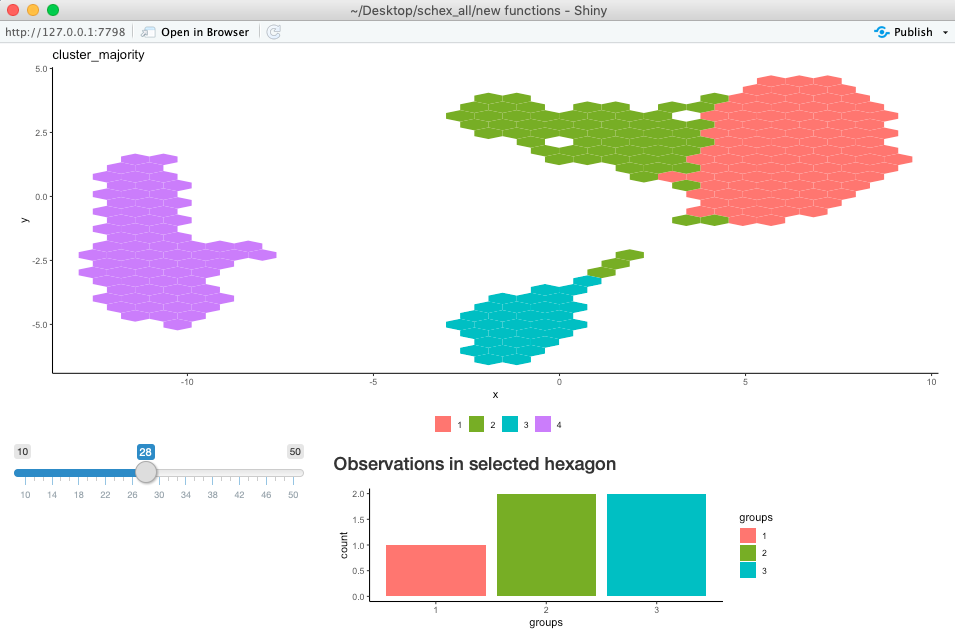# Picking the right resolution

library(igraph)
library(schex)
library(TENxPBMCData)
library(scater)
library(scran)
library(ggrepel)

The resolution of the schex plots is determined by the number of bins the range of the first component of the chosen dimension reduction is partitioned into. Different values of this parameter can result in different looking plots, especially for small datasets. In order to investigate this effect further, schex offers a interactive plot. I will demonstrate the uuse of this plot using the Peripheral Blood Mononuclear Cells (PBMC) freely available from 10x Genomics. I will do minimal processing.

## Setup single cell data

The data is handly availabe in the TENxPBMCData package.

tenx_pbmc3k <- TENxPBMCData(dataset = "pbmc3k")

rownames(tenx_pbmc3k) <- uniquifyFeatureNames(rowData(tenx_pbmc3k)$ENSEMBL_ID, rowData(tenx_pbmc3k)$Symbol_TENx)

### Filtering

I filter cells with high mitochondrial content as well as cells with low library size or feature count.

rowData(tenx_pbmc3k)$Mito <- grepl("^MT-", rownames(tenx_pbmc3k)) colData(tenx_pbmc3k) <- cbind(colData(tenx_pbmc3k), perCellQCMetrics(tenx_pbmc3k, subsets=list(Mt=rowData(tenx_pbmc3k)$Mito)))
rowData(tenx_pbmc3k) <- cbind(rowData(tenx_pbmc3k),
perFeatureQCMetrics(tenx_pbmc3k))

tenx_pbmc3k <- tenx_pbmc3k[, !colData(tenx_pbmc3k)$subsets_Mt_percent > 50] libsize_drop <- isOutlier(tenx_pbmc3k$total,
nmads = 3,type = "lower", log = TRUE)

## Interactively exploring resolution in schex plots

### Exploring changes to density

To see the effect of the resolution parameter on the density, schex offers a handy interactive function. The interactive function opens a shiny application where you can change the resolution parameter using a slider. To find an appropriate value for the resolution parameter find a point where the appearance of the plot is stable.

plot_hexbin_density_shiny(tenx_pbmc3k, 10, 50, dimension_reduction = "UMAP")

### Exploring changes to feature plot

The effect of the resolution parameter on plots of feature expression can also be investigated using an interactive function. In particular, not only can the resolution be directly changed, but also each hexagon can be interrogated. The user can click on a hexagon and will be shown a histogram of the data represented by the histogram.

plot_hexbin_feature_shiny(tenx_pbmc3k, type="counts", feature="POMGNT1",
action="prop_0", min_nbins=10, max_nbins=50, dimension_reduction="UMAP",
mod="RNA")### Exploring changes to meta plot

The effect of the resolution parameter on plots of meta data can also be investigated using an interactive function. In particular, not only can the resolution be directly changed, but also each hexagon can be interrogated. The user can click on a hexagon and will be shown a histogram or bar plot of the data represented by the histogram.

plot_hexbin_meta_shiny(tenx_pbmc3k, col="cluster",
action="majority", min_nbins=10, max_nbins=50, dimension_reduction="UMAP")